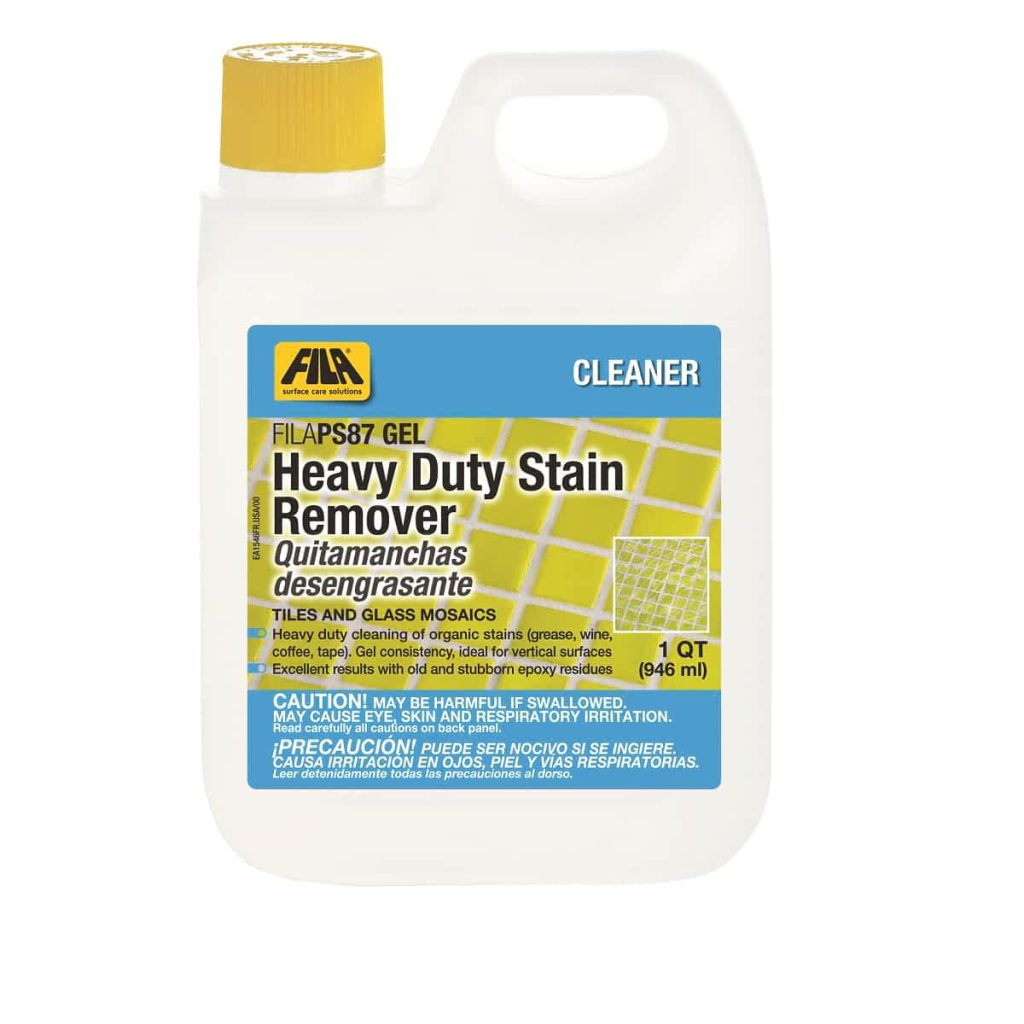# What are the epoxy grout cost per square foot

When the choice of grout for the tiling of a surface is being made, there are several considerations like ease of mixing and application, final look, longevity, effectiveness etc. But another important consideration is the cost of epoxy grout vs regular grout. That is why it is important for you to know how the calculations are made. If you are getting it done, then you could make rough calculations of the raw material requirement. And if you are doing it yourself, you will anyway need to do the calculations accurately.

### How to calculate epoxy grout cost per square foot

Before beginning to calculate the cost of grout per square metre, you need to understand the way tiles are laid. Every tile is of rectangular shape, and tiles are laid one after the other horizontally and one above the other vertically to cover the entire area. So in terms of area, two sets of dimensions would be needed. The first is the overall area to be tiled. You can multiply the length and the breadth of the space to get the area, assuming that the shape of the space to be tiled is a perfect rectangle or square. The second calculation is the area covered by each tile. This is easily calculated by checking the dimensions of the tiles printed on the boxes in which the tiles have been delivered to you. fila grout haze remover

These are the easier part of the calculation. Next you need to understand that the grout will also fill up the gap between two adjacent tiles, which is also called the grout line joint. Also, it must be noted that there are 4 different grout lines for each tile which is at the centre of the tile space, and the calculation must be done for them. For the tiles at the edges of the tile space, each tile might have 2 or 3 grout lines. Depending on where each tile is on the tile space (centre or edge), the number of grout lines will be calculated. Depending on the surface, you would also need to fix the depth (which would depend on the thickness of the tiles) of the grout lines, and also the width of the grout line (gap between successive tiles).

As you can see, there are at least 5 variables in the above calculation, and so a standard formula can’t be provided for calculating the epoxy grout cost per square foot. Let us try to discuss an example using 5 random values of these 5 variables. Let us assume that you are using 5 inch by 2.5 inch tiles in order to cover a tile space of 100 square feet of a rectangular wall. We can consider the standard dimensions of half an inch for the width of grout line and depth of grout line. Using these dimensions, the requirement would be for approximately 2160 cubic inches of grout mortar. Converting this to standard dimensions, you can assume a requirement of 1.25 cubic feet. Considering a coverage of 3 cubic feet for every 10 units of a standard epoxy mix, we can calculate that around 4.17 units would be needed, which means you would need to purchase 5 units.